Examples

Chapter 10 Class 10 Circles
Serial order wise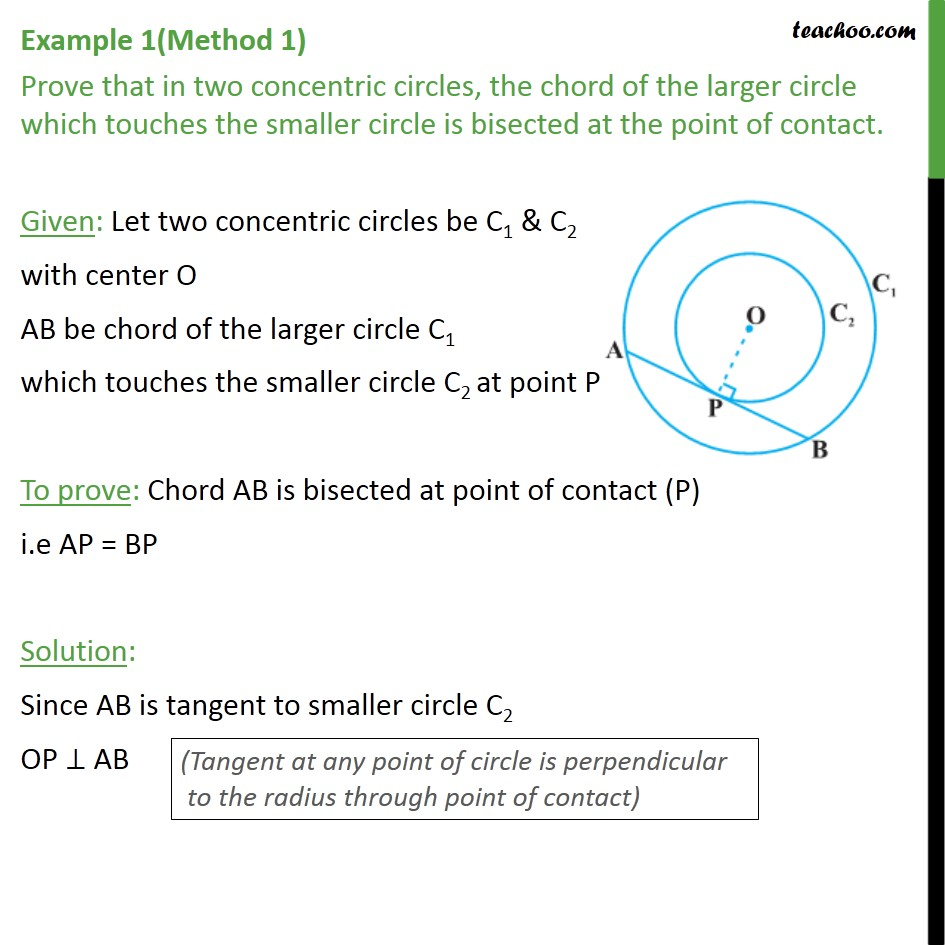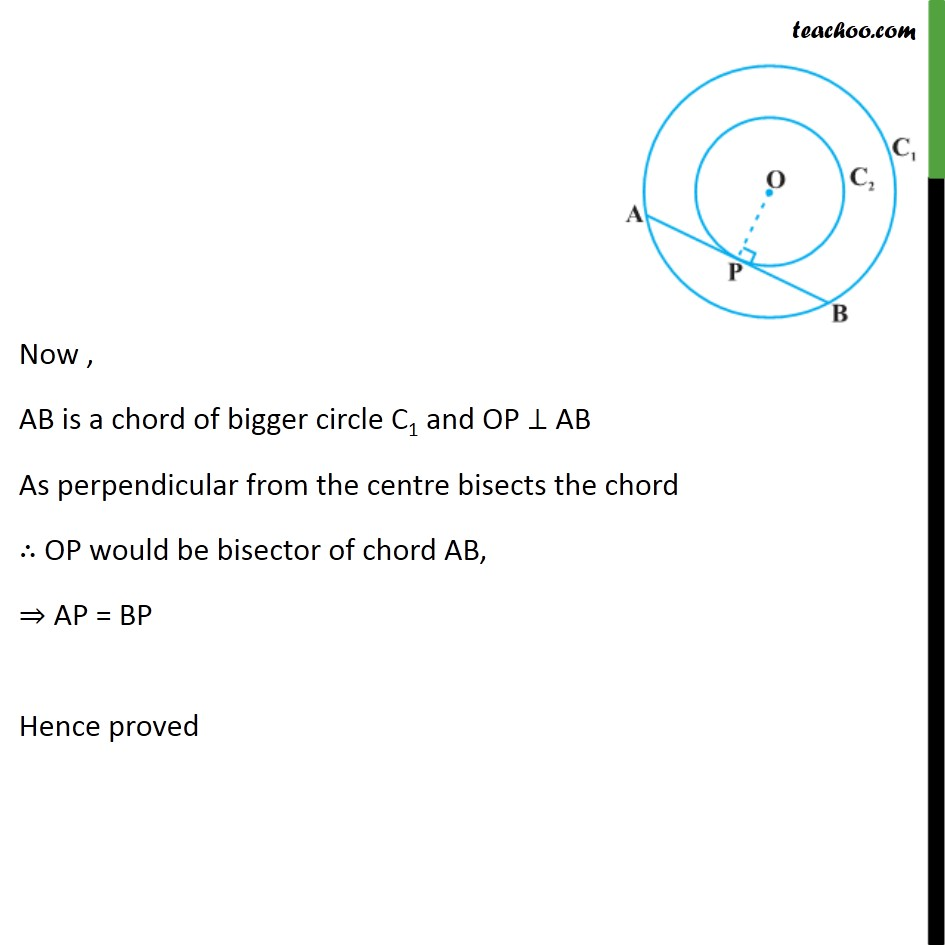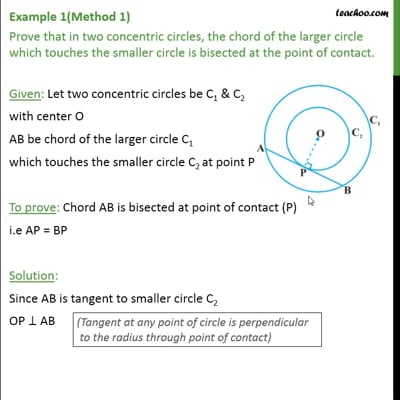This video is only available for Teachoo black users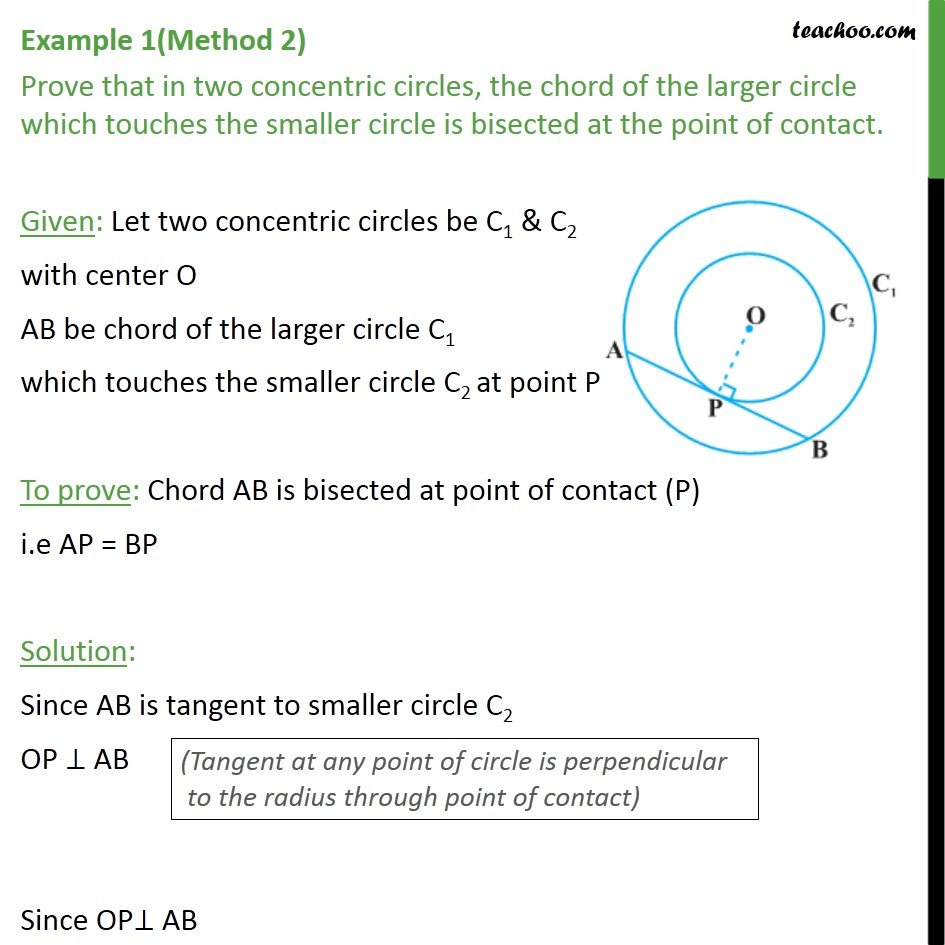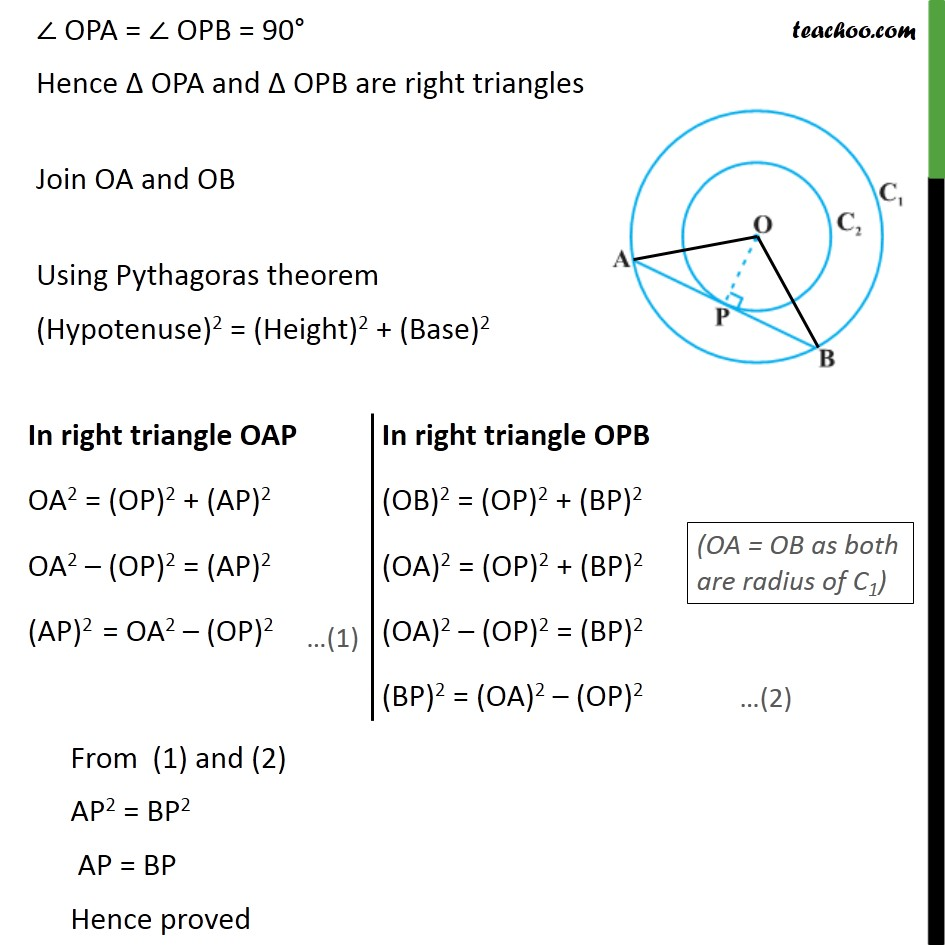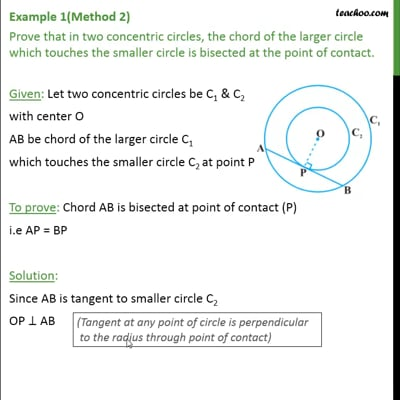This video is only available for Teachoo black users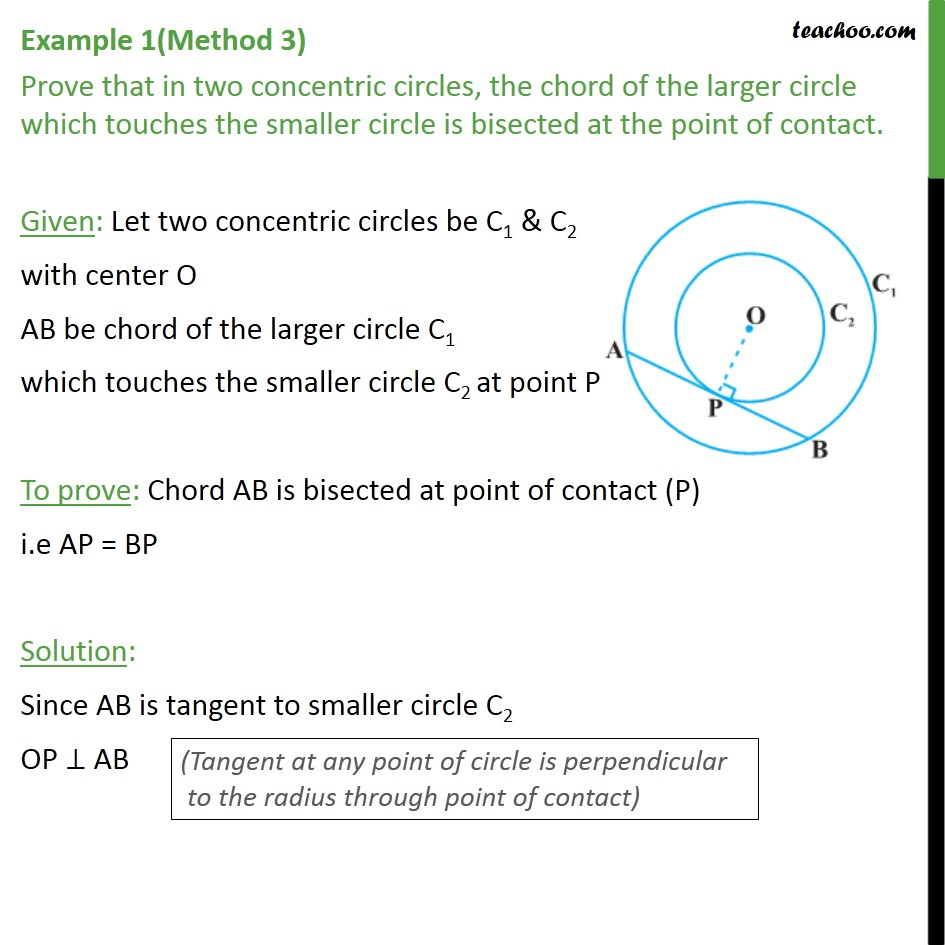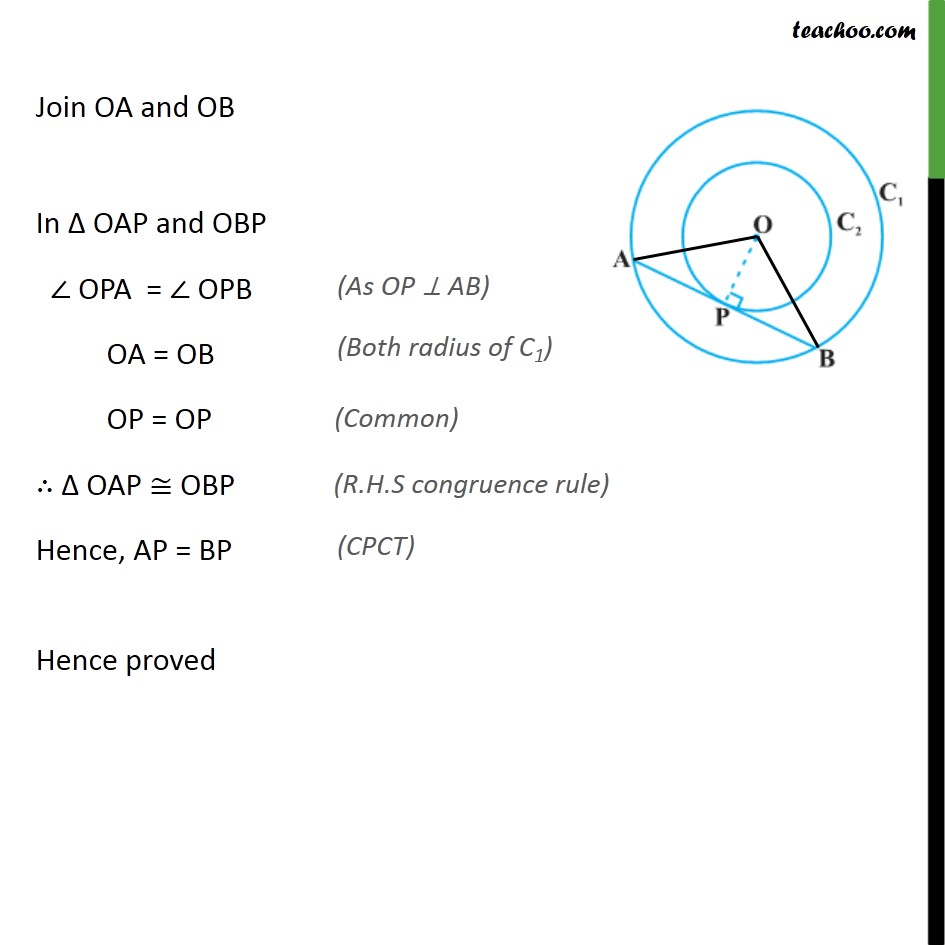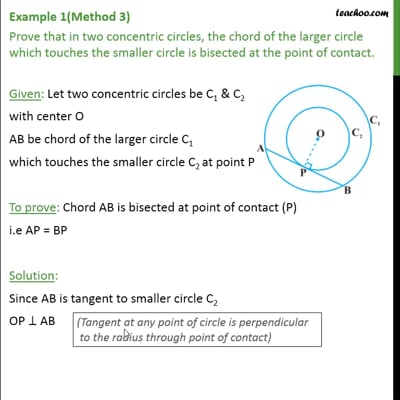This video is only available for Teachoo black users

Solve all your doubts with Teachoo Black (new monthly pack available now!)

### Transcript

Example 1(Method 1) Prove that in two concentric circles, the chord of the larger circle which touches the smaller circle is bisected at the point of contact. Given: Let two concentric circles be C1 & C2 with center O AB be chord of the larger circle C1 which touches the smaller circle C2 at point P To prove: Chord AB is bisected at point of contact (P) i.e AP = BP Solution: Since AB is tangent to smaller circle C2 OP ⊥ AB Now , AB is a chord of bigger circle C1 and OP ⊥ AB As perpendicular from the centre bisects the chord ∴ OP would be bisector of chord AB, ⇒ AP = BP Hence proved Example 1(Method 2) Prove that in two concentric circles, the chord of the larger circle which touches the smaller circle is bisected at the point of contact. Given: Let two concentric circles be C1 & C2 with center O AB be chord of the larger circle C1 which touches the smaller circle C2 at point P To prove: Chord AB is bisected at point of contact (P) i.e AP = BP Solution: Since AB is tangent to smaller circle C2 OP ⊥ AB Since OP⊥ AB ∠ OPA = ∠ OPB = 90° Hence Δ OPA and Δ OPB are right triangles Join OA and OB Using Pythagoras theorem (Hypotenuse)2 = (Height)2 + (Base)2 Example 1(Method 3) Prove that in two concentric circles, the chord of the larger circle which touches the smaller circle is bisected at the point of contact. Given: Let two concentric circles be C1 & C2 with center O AB be chord of the larger circle C1 which touches the smaller circle C2 at point P To prove: Chord AB is bisected at point of contact (P) i.e AP = BP Solution: Since AB is tangent to smaller circle C2 OP ⊥ AB Join OA and OB In Δ OAP and OBP ∠ OPA = ∠ OPB OA = OB OP = OP ∴ Δ OAP ≅ OBP Hence, AP = BP Hence proved